Homework Help Question & Answers

# What coefficient of friction is needed to keep the 5-kg block A from sliding on the...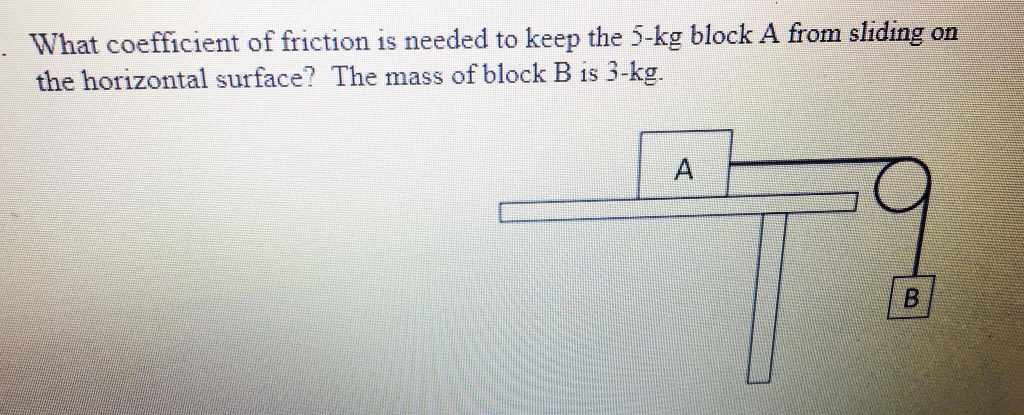What coefficient of friction is needed to keep the 5-kg block A from sliding on the horizontal surface? The mass of block B is 3 -kg.

#### Homework Answers

Answer #1

Let the Coefficient of kinetic friction be uk .

Tension in the string, T = 3.0 * 9.8 = 29.4 N

For Block A not to slide,
Fr = T
uk*5.0*9.8 = 29.4
uk = 0.6

Know the answer?
Your Answer:

#### Post as a guest

Your Name:

What's your source?

#### Earn Coin

Coins can be redeemed for fabulous gifts.

Not the answer you're looking for? Ask your own homework help question. Our experts will answer your question WITHIN MINUTES for Free.
Similar Homework Help Questions
• ### The coefficient of kinetic friction for a block of mass 45 kg sliding down an inclined...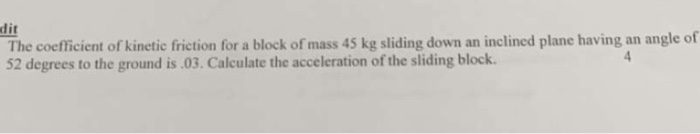The coefficient of kinetic friction for a block of mass 45 kg sliding down an inclined plane having an angle of 52 degrees to the ground is .03. Calculate the acceleration of the sliding block.

• ### 1. A sliding block of mass m-0.25 kg is subject to a force of magnitude 4...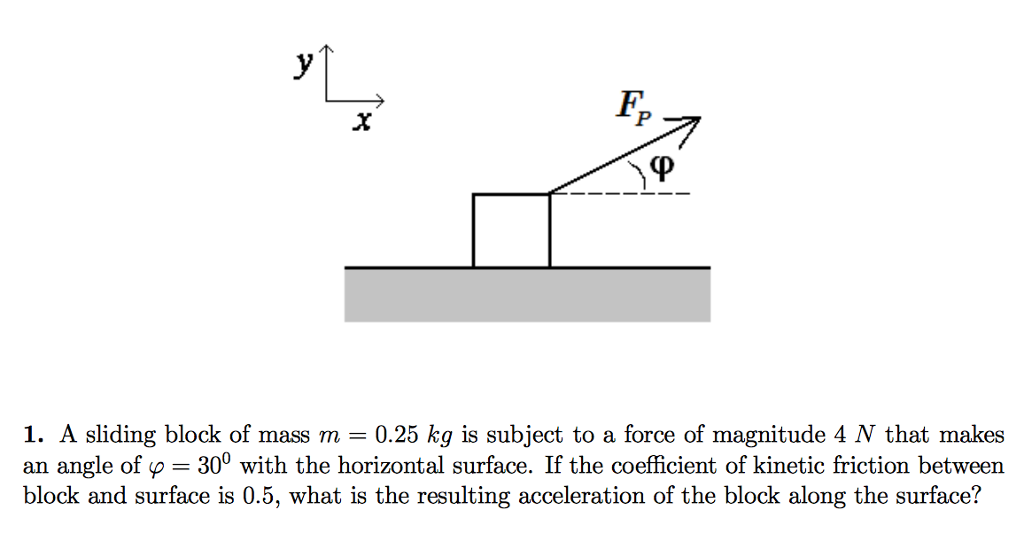1. A sliding block of mass m-0.25 kg is subject to a force of magnitude 4 N that makes an angle of p 30° with the horizontal surface. If the coefficient of kinetic friction between block and surface is 0.5, what is the resulting acceleration of the block along the surface?

• ### Questions 1&2 FP 1. A sliding block of mass m 0.25 kg is subject to a...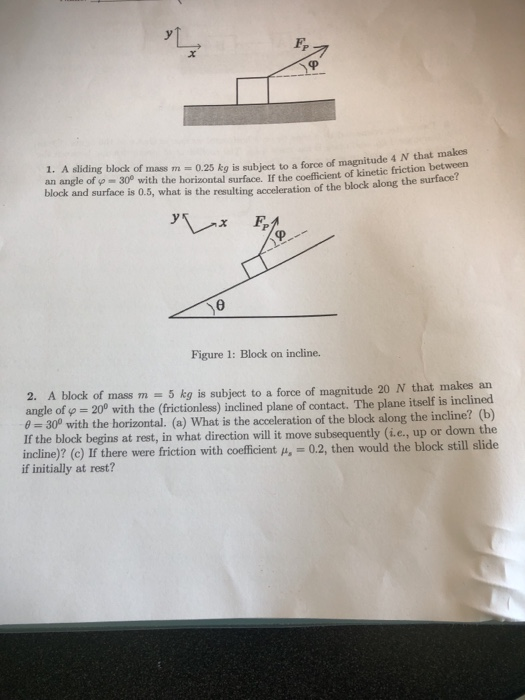Questions 1&2 FP 1. A sliding block of mass m 0.25 kg is subject to a force of magnitude 4 N that makes an angle of ф-30 with the horizontal surface. If the coefficient of kinetic friction between block and surface is 0.5, what is the resulting acceleration of the block along the surface Figure 1: Block on incline. 2. A block of mass m - 5 kg is subject to a force of magnitude 20 N that makes an...

• ### A 5 kg block rests on a 30 degree incline. The coefficient of static friction between...

A 5 kg block rests on a 30 degree incline. The coefficient of static friction between the block and the incline is .20. How large a horizontal force must push on the block if the block is to be on the verge of sliding A) up the incline and B) down an incline Answer: A) 43 N B) 16.6 N What would be the work to get me to this answer?

• ### m. A block with mass m, = 9.4 kg is on an incline with an angle...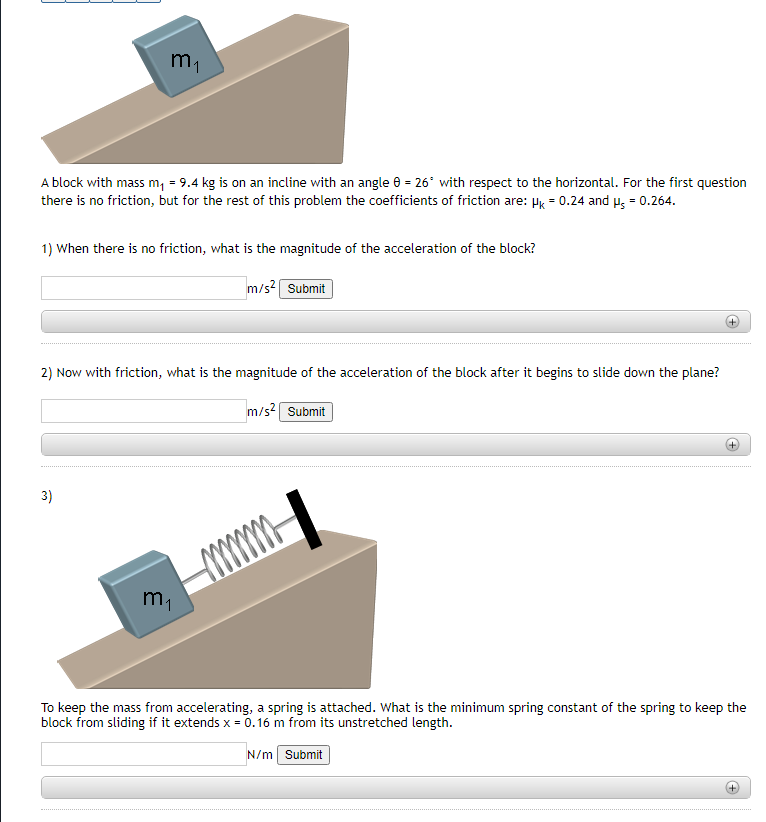m. A block with mass m, = 9.4 kg is on an incline with an angle 6 = 26" with respect to the horizontal. For the first question there is no friction, but for the rest of this problem the coefficients of friction are: Pk = 0.24 and ys = 0.264. 1) When there is no friction, what is the magnitude of the acceleration of the block? m/s2 Submit 2) Now with friction, what is the magnitude of the acceleration...

• ### A 2 kg block is sliding down a plane inclined at 30˚ to the horizontal. The...

A 2 kg block is sliding down a plane inclined at 30˚ to the horizontal. The coefficient of friction           between the block and the plane is 0.25. How much work is done by gravity if the block slides 5 m?           How much work is done by friction? If the block starts from rest, what is its speed after sliding the           5 m?

• ### A block with a mass of 4 kg is sliding across a horizontal surface with an...

A block with a mass of 4 kg is sliding across a horizontal surface with an initial speed of 5 m/s. Because of kinetic friction, the energy of this system will decrease linearly with the distance travelled by the block. a. What is the magnitude of the force of kinetic friction (in N) acting on the block if the block moves 4 m before coming to a stop? b. What is the average power (in W) supplied by the force...

• ### m A block with mass m, - 8.9 kg is on an incline with an angle...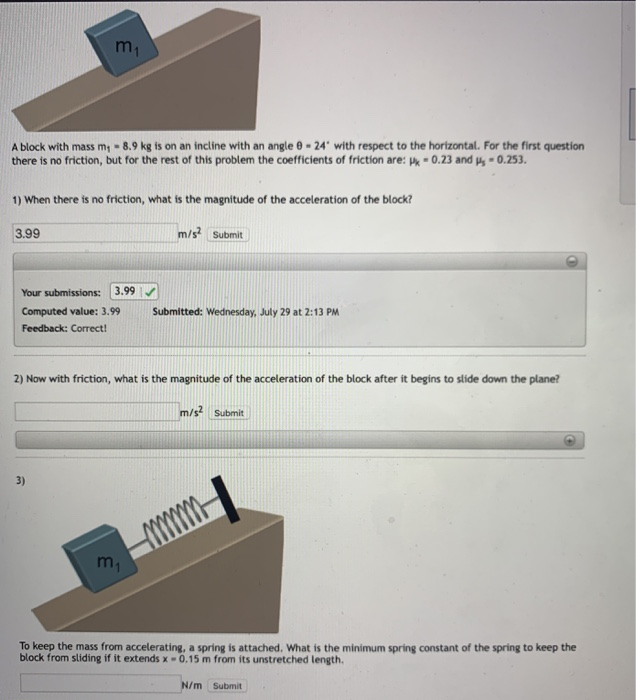m A block with mass m, - 8.9 kg is on an incline with an angle 8 - 24" with respect to the horizontal. For the first question there is no friction, but for the rest of this problem the coefficients of friction are: Pk - 0.23 and Ms - 0.253. 1) When there is no friction, what is the magnitude of the acceleration of the block? 3.99 m/s2 Submit Your submissions: 3.99 Computed value: 3.99 Feedback: Correct! Submitted: Wednesday,...

• ### Friction coefficient of friction support force, sliding or static coefficient Three horizontally stacked blocks was pushed...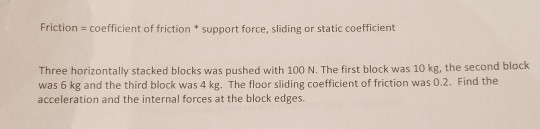Friction coefficient of friction support force, sliding or static coefficient Three horizontally stacked blocks was pushed with 100 N. The first block was 10 kg, the second block was 6 kg and the third block was 4 kg. The floor sliding coefficient of friction was 0.2. Find the acceleration and the internal forces at the block edges.

• ### C. A 15 0-kg box has a rubber bottom. It is sliding on a horizontal sheet...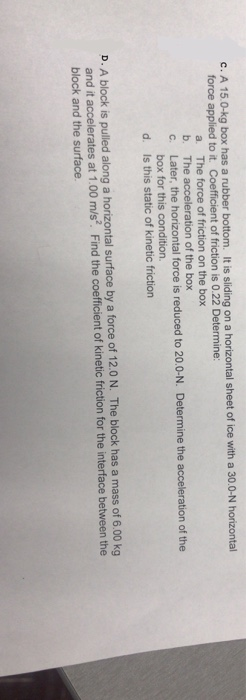C. A 15 0-kg box has a rubber bottom. It is sliding on a horizontal sheet of ice with a 30.0 force applied to it. Coefficient of friction is 0.22 Determine: a. The force of friction on the box The acceleration of the box c. Later, the horizontal force is reduced to 20.0-N. Determine the acceleration of the box for this condition. d. Is this static of kinetic friction D. A block is pulled along a horizontal surface by a...

Free Homework App

Scan Your Homework
to Get Instant Free Answers
Need Online Homework Help?

Get Answers For Free
Most questions answered within 3 hours.